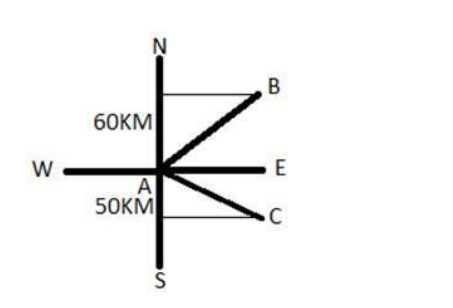# Two boats leave a port at the same time.`
Question:

Two boats leave a port at the same time. One travels 60 km in the direction N 500 E while the other travels 50 km in the direction S 700 E. What is the distance between the boats?

Solution:Both the boats starts from $A$ and boat 1 reaches at $B$ and boat 2 reaches at $C$.

Here, $A B=60 \mathrm{Km}$ and $A C=50 \mathrm{Km}$

So, the net distance between ta boats is:

$|\overrightarrow{\mathrm{BC}}|=|\overrightarrow{\mathrm{AC}}-\overrightarrow{\mathrm{AB}}|$

$=\sqrt{60^{2}+50^{2}-2.60 .50 \cdot \cos 60^{0}}$

$=\sqrt{3600+2500-3000}$

$=55.67 \mathrm{Km}$